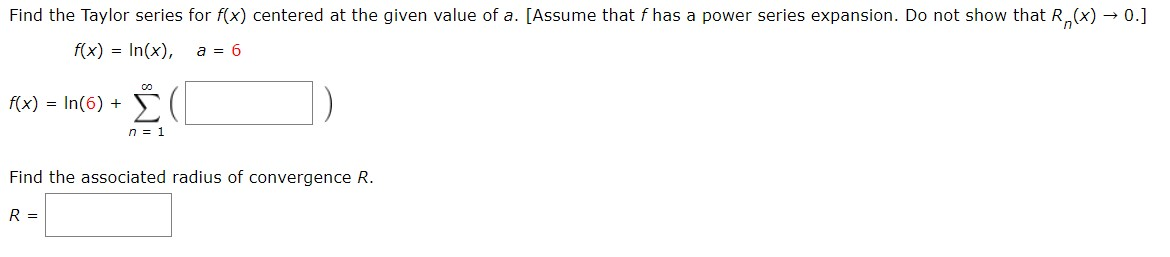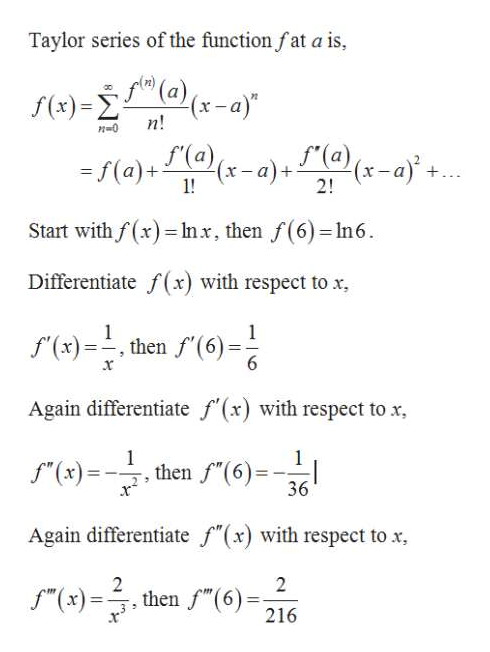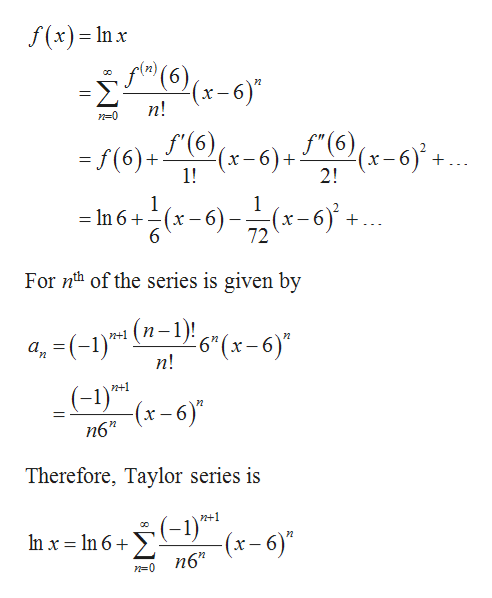# Find the Taylor series for f(x) centered at the given value of a. [Assume that f has a power series expansion. Do not show that R(x) 0.]f(x) In(x)a= 6coΣf(x) In(6) +n1Find the associated radius of convergence R.R =

Question
18 viewshelp_outlineImage TranscriptioncloseFind the Taylor series for f(x) centered at the given value of a. [Assume that f has a power series expansion. Do not show that R(x) 0.] f(x) In(x) a= 6 co Σ f(x) In(6) + n1 Find the associated radius of convergence R. R = fullscreen
check_circle

Step 1

From the given statement, the function f (x) = ln(x), a = 6.help_outlineImage TranscriptioncloseTaylor series of the function fat a is (a)(x-a) n! S()x-a)a (x-a f(a)+ + 1! 2! Start with f(x) Inx, then f(6)-In6. Differentiate f(x) with respect to x, 1 f(x)thenf(6) 6 x Again differentiate f(x) with respect to x 1 then f"(6) 1 (x)- 36 Again differentiate f"(x) with respect to x 2 ,then f"(6) 2 (x)=t =- 216 fullscreen
Step 2

From this, Taylor se...help_outlineImage Transcriptionclosef(x)= Inx f(6 (x-6 п! S"(6 (x-6+ F(x-6) (6)+(6) 1! 2! 1 -6 =In 6+(x-6)2 +. х 6 72 For nth of the series is given by (-1)*8 а, п! (-1)T* -(x-6)" n+1 Therefore, Taylor series is n+1 In x n 6 -(x-6)" n6" n0 fullscreen

### Want to see the full answer?

See Solution

#### Want to see this answer and more?

Solutions are written by subject experts who are available 24/7. Questions are typically answered within 1 hour.*

See Solution
*Response times may vary by subject and question.
Tagged in